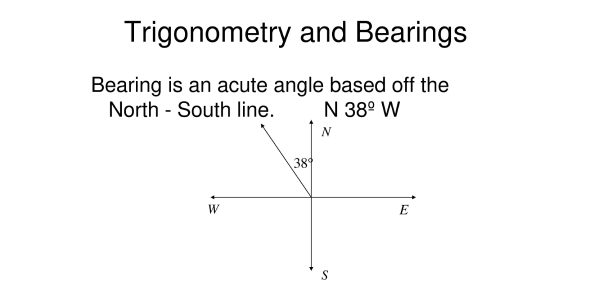# Bearings In Trigonometry! Trivia Quiz

10 Questions | Attempts: 1587
ShareSettings.

• 1.
For the above diagram,find the bearing of A from OYou do not have to write the word "degrees"
• 2.
From the previous question, find the bearing of C from OYou do not have to write the word "degrees"
• 3.
From the previous question, find the bearing of O from A You do not have to write the word "degrees"
• 4.
From the previous question, find the bearing of O from D You do not have to write the word "degrees"
• 5.
From the previous question, find the bearing of B from North You do not have to write the word "degrees"
• 6.
In the diagram above, AB = 10cm, angle CAB = 70 degrees and angle ABC = 34 degreesFind the bearing of B from C
• 7.
From the previous question,Find the bearing of A from C
• 8.
From the previous question,Find the length of the line, from A, which is perpendicular to BCLeave your answer to three significant figuresRemember to put "cm"
• 9.
For the diagram above, the bearing of C from A is 045 degrees and the bearing of C from B is 015 degrees. AB=BC=20cm. The bearing of B from A is 075 degrees.Find the length of the line from B which is perpendicular to ACRemember to put "cm"
• 10.
From the previous question, Find the length of ACLeave your answer to three significant figuresRemember to put "cm"

## Related TopicsBack to top
×

Wait!
Here's an interesting quiz for you.